## Fourier Series

Fourier series are expansions of Periodic Functionsin terms of an infinite sum of Sines and Cosines(1)

Fourier series make use of the Orthogonality relationships of the Sine and Cosine functions, which can be used to calculate the coefficients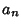andin the sum. The computation and study of Fourier series is known as Harmonic Analysis.

To compute a Fourier series, use the integral identities(2)(3)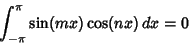(4)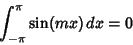(5)(6)

whereis the Kronecker Delta. Now, expand your functionas an infinite series of the form(7)

where we have relabeled the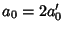term for future convenience but setand leftfor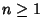. Assume the function is periodic in the interval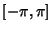. Now use the orthogonality conditions to obtain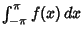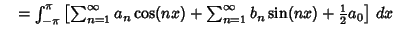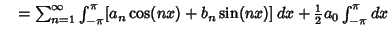(8)
and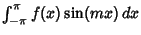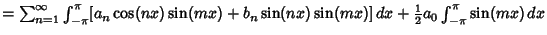(9)
so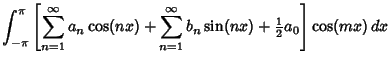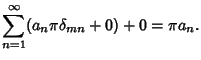(10)

Plugging back into the original series then gives(11)(12)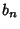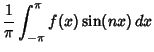(13)

for, 2, 3, .... The series expansion converges to the function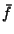(equal to the original function at points of continuity or to the average of the two limits at points of discontinuity)(14)

if the function satisfies the Dirichlet Conditions.Near points of discontinuity, a ringing'' known as the Gibbs Phenomenon, illustrated above, occurs. For a functionperiodic on an interval, use a change of variables to transform the interval of integration to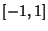. Let(15)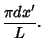(16)

Solving for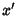,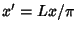. Plugging this in gives(17)(18)

If a function is Even so that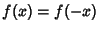, then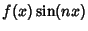is Odd. (This follows sinceis Odd and an Even Function times an Odd Function is an Odd Function.) Therefore,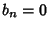for all. Similarly, if a function is Odd so that, thenis Odd. (This follows sinceis Even and an Even Function times an Odd Function is an Odd Function.) Therefore,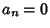for all.

Because the Sines and Cosines form a Complete Orthogonal Basis, the Superposition Principle holds, and the Fourier series of a linear combination of two functions is the same as the linear combination of the corresponding two series. The Coefficients for Fourier series expansions for a few common functions are given in Beyer (1987, pp. 411-412) and Byerly (1959, p. 51).

The notion of a Fourier series can also be extended to Complex Coefficients. Consider a real-valued function. Write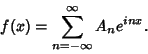(19)

Now examine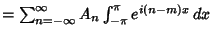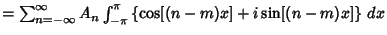(20)
so(21)

The Coefficients can be expressed in terms of those in the Fourier Series(22)

For a function periodic in, these become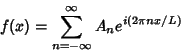(23)(24)

These equations are the basis for the extremely important Fourier Transform, which is obtained by transformingfrom a discrete variable to a continuous one as the length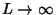.

See also Dirichlet Fourier Series Conditions, Fourier Cosine Series, Fourier Sine Series, Fourier Transform, Gibbs Phenomenon, Lebesgue Constants (Fourier Series), Legendre Series, Riesz-Fischer Theorem

References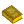Fourier Transforms

Arfken, G. Fourier Series.'' Ch. 14 in Mathematical Methods for Physicists, 3rd ed. Orlando, FL: Academic Press, pp. 760-793, 1985.

Beyer, W. H. (Ed.). CRC Standard Mathematical Tables, 28th ed. Boca Raton, FL: CRC Press, 1987.

Brown, J. W. and Churchill, R. V. Fourier Series and Boundary Value Problems, 5th ed. New York: McGraw-Hill, 1993.

Byerly, W. E. An Elementary Treatise on Fourier's Series, and Spherical, Cylindrical, and Ellipsoidal Harmonics, with Applications to Problems in Mathematical Physics. New York: Dover, 1959.

Carslaw, H. S. Introduction to the Theory of Fourier's Series and Integrals, 3rd ed., rev. and enl. New York: Dover, 1950.

Davis, H. F. Fourier Series and Orthogonal Functions. New York: Dover, 1963.

Dym, H. and McKean, H. P. Fourier Series and Integrals. New York: Academic Press, 1972.

Folland, G. B. Fourier Analysis and Its Applications. Pacific Grove, CA: Brooks/Cole, 1992.

Groemer, H. Geometric Applications of Fourier Series and Spherical Harmonics. New York: Cambridge University Press, 1996.

Körner, T. W. Fourier Analysis. Cambridge, England: Cambridge University Press, 1988.

Körner, T. W. Exercises for Fourier Analysis. New York: Cambridge University Press, 1993.

Lighthill, M. J. Introduction to Fourier Analysis and Generalised Functions. Cambridge, England: Cambridge University Press, 1958.

Morrison, N. Introduction to Fourier Analysis. New York: Wiley, 1994.

Sansone, G. Expansions in Fourier Series.'' Ch. 2 in Orthogonal Functions, rev. English ed. New York: Dover, pp. 39-168, 1991.